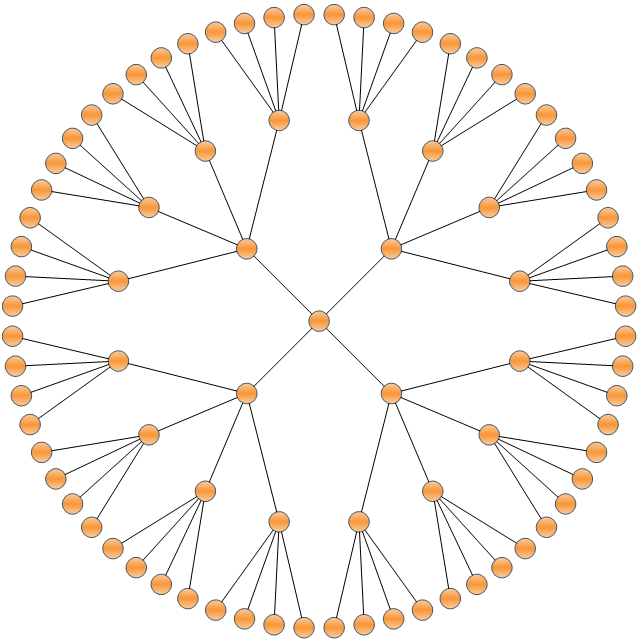Nevron .NET Vision Documentation

The radial graph layout is represented by the NRadialGraphLayout class. The radial graph layout algorithm layouts the graph in concentric circles. The vertices with no predecessors are placed in the center and their descendants are placed on the next circle and so on. It produces a straight line graph drawing.

• AspectRatio - determines the aspect (width/height) ratio of the layout. By default set to 1 which layouts the nodes in a circle. A value different from 1 will make the layout order the nodes in an ellipse.
• AutoSizeRings - if set to true the RingRadius property is automatically calculated to have such value that the total area of the drawing is minimized and there is no node overlapping.
• RingRadius - determines the size of the radius of the first imaginary circle where nodes are placed. The radius of each other circle is a sum of the RingRadius value and the radius of the previous circle. This value is automatically determined if the AutoSizeRings property is set to true.

The following image demonstrates the result of the algorithm for a tree graph.Related Examples

Windows Forms: Layouts - Radial Graph Layout

Web Forms: Layouts - Radial Graph Layout